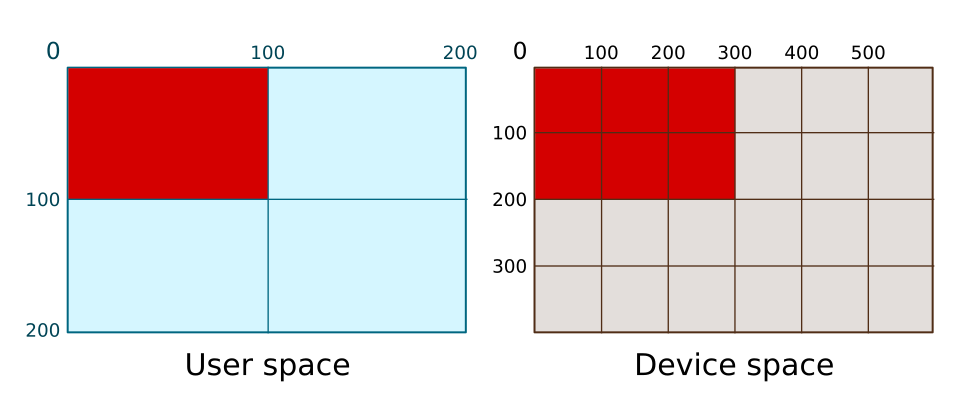# Transforms in Pycairo - scale

Martin McBride, 2019-09-27
Tags transform scaling user space device space
Categories pycairoWe have previously seen how to use translate in Pycairo, to draw items in different positions on the page. In this article we will see how to use scaling to draw items at different sizes.

## Default user space

We will start once again by drawing a shape in default user space, with no transform applied.

ctx.rectangle(0, 0, 100, 100)
ctx.set_source_rgb(0, 0, 1)
ctx.fill()


This defines the rectangle in user space. Pycairo then draws the square in device space. Because of the default mapping, the square is drawn at exactly the same size and place in device space:## Scaling

The scale function scales user space. Here is an example:

ctx.scale(2, 2)
ctx.rectangle(0, 0, 100, 100)
ctx.set_source_rgb(0, 0, 1)
ctx.fill()


This scales user space by a factor of 2. Notice that scale takes 2 arguments. They control scaling in the x and y directions. Set them bot to the same value to have the same scaling in all directions.

Here is how the square is drawn using the scaled user space:Notice that the scale of user space is doubled, but device space remains unchanged (as it always does). So now when we draw a square that is 100 units wide (in user space) the actual square that gets drawn in device space is 200 units wide. Not surprisingly, a scale factor of 2 draws a square that is twice as big.

## Unequal scaling

We can set the x and y scale factors to different values in the scale function, like this:

ctx.scale(3, 2)
ctx.rectangle(0, 0, 100, 100)
ctx.set_source_rgb(0, 0, 1)
ctx.fill()


This scales user space by a factor of 3 in the x direction, and 2 in the y direction.

Here is how the square is drawn using the scaled user space:Notice that the user space grid is no longer square! 100 user units in the x direction have been scaled to be equal to 300 units in device space, but 100 user units in the y direction are equal to 200 units in device space. So when we draw a 100 unit square in user space, what we actually draw on the canvas is a rectangle of 300 by 100 pixels.

If you found this article useful, you might be interested in the book Computer Graphics in Python or other books by the same author.

#### Popular tags

2d arrays abstract data type alignment and angle animation arc array arrays bar chart bar style behavioural pattern bezier curve built-in function callable object chain circle classes clipping close closure cmyk colour combinations comparison operator comprehension context context manager conversion count creational pattern data science data types decorator design pattern device space dictionary drawing duck typing efficiency ellipse else encryption enumerate fill filter font font style for loop function function composition function plot functools game development generativepy tutorial generator geometry gif global variable gradient greyscale higher order function hsl html image image processing imagesurface immutable object in operator index inner function input installing iter iterable iterator itertools join l system lambda function len lerp line line plot line style linear gradient linspace list list comprehension logical operator lru_cache magic method mandelbrot mandelbrot set map marker style matplotlib monad mutability named parameter numeric python numpy object open operator optimisation optional parameter or pandas partial application path pattern permutations pie chart polygon positional parameter print pure function python standard library radial gradient range recipes rectangle recursion reduce regular polygon repeat rgb rotation roundrect scaling scatter plot scipy sector segment sequence setup shape singleton slice slicing sound spirograph sprite square str stream string stroke structural pattern subpath symmetric encryption template text text metrics tinkerbell fractal transform translation transparency triangle truthy value tuple turtle unpacking user space vectorisation webserver website while loop zip